This document details the process of creating, assigning, and running SLO Formulas using the associated pages within RightPath.

Creating and Applying SLO Formulas (Calculate Targets & Post-Exam Scores)

The SLO Goal Form captures student achievement using baseline, target, and summative score data. Within RightPath™ the students’ targets and summative exam scores can now be calculated, manipulated, and applied comprehensively using SLO Formulas. Formulas can reference multiple exams, include conditional logic, and be applied to templates and individual SLOs. The tool’s ability to extract data from various sources allows for target calculation utilizing an exams’ summative score data from the preceding school-year as the baseline score value.

The SLO Formulas feature can generate virtually any desired calculation in order to manage multiple student populations, applicable exam or target adjustments, and other necessary scoring criteria all within a single ruleset. The page includes a simple user-friendly design and allows formula entries to be saved at any time for later editing.

Creating SLO Formulas

Once the SLO Formulas permission has been enabled for your RightPath™ user account, adding and editing SLO Formulas consists of the following simple steps: navigating to the SLO Formulas Search page and creating a new formula and its application type.

1. The SLO Formulas page is accessed within the SLOs menu from the drop-down APPR tab anywhere within RightPath™.2. To begin working on a new formula, click the "Create Formula" button located above the search grid.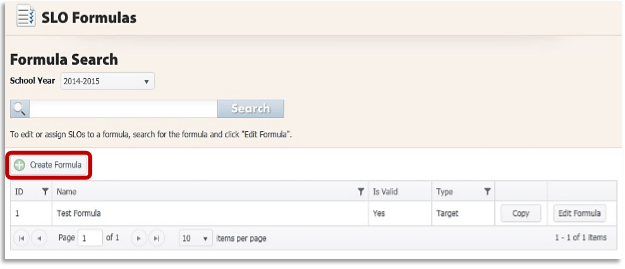3. Complete the required fields for the formula: create a name, select the type of application, and select the appropriate school year. Then, click the "Create" button in order to launch the formula edit screen.Note: Each SLO Formula can output figures in a single SLO Roster column: students’ Target scores and EOY Exam scores will require separate formulas.

Configuring SLO Formulas
The main Edit Formula page is where all formula construction and modification will take place. An array of available actions (rules) are selected to create the steps of each formula in order to arrive at the desired output value for Targets or EOY Exam scores. These include, but are not limited to: Score Lookup, Add/Subtract/Multiply/Divide/Average Two Values, Get Constant/Test Score, Condition, and If-Then Return.1 Formulas must reference previous steps’ Output values. Any required piece of a Formula must appear as a step’s Output to be utilized in a subsequent calculation with constants or conditions.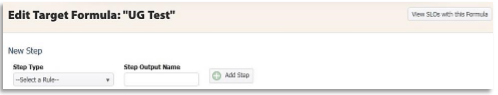1. The Formula rules must reference a previous step in the sequence. Most Targets will refer to a previously uploaded Baseline score in their calculations. In these cases the SLO Formula will require the first step to be Get Test Score, which will access the SLO Exam Catalog so that the desired exam can be selected. Title the Output value, then click the "Add Step" button to launch the associated "Exam Search" pop-up.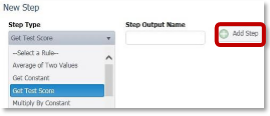2. Start by choosing a source for data using the Get Test Score step. Filter the school year and exam type (Pre or Post), choose the desired exam from the populated list of results, and click the "Save" button. The step will be added to the Edit SLO Formula page and the green “Success” message will appear.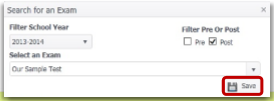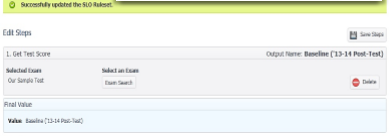Each new Rule acts upon the output of one or more preceding steps. Our Get Test Score Rule yields the output value “Baseline,” consisting of students’ scores on “Our Sample Test” (last year’s Post-Exam in the corresponding discipline). Essentially, the tool “gets” the desired Baseline for our Target calculation.

3. Another step in the Target Formula can now be added. If the sample student population uses the preceding school year’s PostExam as the “Baseline” value, and the Target will be a “Growth of 10%”; then the Multiply By Constant Rule as the next step will provide an output value of 10% of the Baseline score.4. Using this ruleset, the final step needed in order to arrive at the Target score will be to obtain a sum. Using the Rule Add Two Values, two output values (the “Baseline” score and the “10% Growth” value) are selected and summed together achieving a final output: the Target score.Note: Steps in the Formula will appear red until they have been saved, or until another step has been added. Any step remaining in red after clicking the "Save Steps" button at the top of the sequence indicates an incalculable action that must be addressed.

Tip: Steps within the SLO Formulas can be re-ordered to address incorrect sequences, add calculations, etc. If a step is missing, it can be added at the end of the sequence and then dragged into its appropriate order within the list of operations.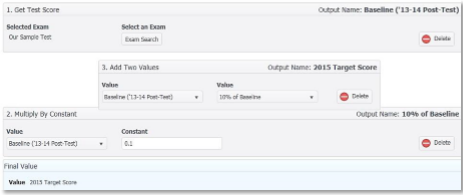Assigning SLO Formulas to SLOs and SLO Templates

Once a SLO Formula is complete, it can be assigned to SLO templates and individual SLOs created from templates. Assigning a SLO Formula to individual SLOs can be completed from both the SLO Formula Edit and the SLO Bulk Assign pages. Assigning a SLO Formula to SLO templates, however, must be completed from the SLO Bulk Assign page. The process requires only a few steps from either location and results in an attached Formula that Target scores, or Post-Exam scores can be calculated from individually, or en masse.

1. SLO Formulas can be assigned from the SLO Formula Edit page by clicking the "View SLOs with this Formula" button. A list of SLOs that are currently using the Formula will populate in the table (if this Formula is new, the table will be empty). The "Assign SLOs" button appears at the top right portion of this page. This button will launch a search grid where SLOs and SLO templates can be located by ID number, title, or keywords.2. Once the results are narrowed down, multiple SLOs can be selected using their accompanying checkboxes in the leftmost column of the search grid. After SLOs are selected, the SLO Formula is assigned by clicking the "Assign Target Formula to SLOs" button. The Formula is assigned and the SLOs are added to the “SLOs with Formula: _____” page. From here, the Assign SLOs page can be reopened for additional SLO linking, or the Formula Edit page can be returned to using the ‘Return to Formula Edit’ navigation arrow highlighted above.Note: A single page of SLOs can be assigned the SLO Formula at once. For this reason, the results page size should be expanded to display the maximum number of results in order to select all SLOs for assigning on one page. If results to be assigned to a Formula span multiple pages, the "Assign Target Formula to SLOs" button should be clicked once for each full page of results. If the SLOs are tied to a SLO template, then the SLO Bulk Assign page should be used for attaching the Formula to the associated SLO template(s).

Bulk Assigning Formulas to SLO Templates

SLO templates are assigned SLO Formulas using the SLO Bulk Assign page (contact a Client Services Representative at RRT for access Permissions). SLOs can be assigned a Formula at the template level, or individual level from this management page. The SLO Formula calculations can also be run and applied to all associated templates and SLO Forms from this page.

1. The SLO Bulk Assign page is accessible from the SLOs menu under the APPR tab from anywhere within RightPath™. Select the “By Template” page option.2. Use the keyword search to narrow the list of available SLO templates. Select the desired template(s) within the search grid using the selection checkboxes in the grid’s leftmost column. SLO Formulas can be assigned, or have calculations run and applied using the Actions menu located above the search grid.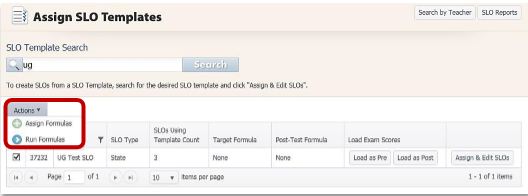3. The "Assign Formulas" menu option will load the Assign SLO Formulas page. The selected template will display in the results table where Target, or Post-test SLO Formulas can be assigned. Use the corresponding checkboxes to apply one or both Formula types: selection menus display all valid options for either type. Click "Assign Formulas" when all necessary fields are addressed. The SLO Formula Assign page will return a list of successful SLO Formula assignments: all affected templates and

associated SLOs will be displayed in this list. From this page the ‘Return to Select’ navigation arrow returns the SLO Bulk Assign page where the SLO Formula calculations can be run.Running SLO Formulas

SLO Formulas are also run using the Actions menu above the Template Search grid on the SLO Bulk Assign page (APPR > SLOs > Bulk Assign SLOs > By Template).

1. Select the checkbox to the left of the desired SLO template and choose the "Run Formulas" option to launch the Run SLO Formulas page. Select the Formula type(s), and click "Run Formulas".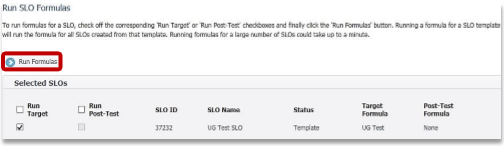2. The Run Formula Results page displays the calculation outcomes for each associated SLO during the current Formula Run.3. The "Details" button displays a table with additional information on the outcome of the SLO Formula Run: including the status of successful SLO Formula runs, and the reason for failures.4. The "Results" button is available in the table for successful SLO Formula runs. It will launch a popup window with an expanded view of the SLO Formula calculations for each student on a SLO.